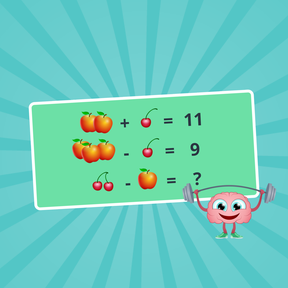Brain Breaks: Math Puzzles

# Brain Breaks: Math Puzzles

Challenge your math knowledge with these puzzles.8,000 schools use Gynzy92,000 teachers use Gynzy1,600,000 students use Gynzy

## General

Students practice their problem-solving skills with these challenging math puzzles! Each fruit or vegetable represents a number. Find the value of the individual fruits or vegetables by solving the mathematical equations.
Choose from three options: addition & subtraction, adding, subtracting, multiplying, and dividing, or go for the extra challenging problems that require students to solve for all the fruit or vegetables rather than one.
These pre-algebraic puzzles that use substitution help students perform basic functions and encourage problem-solving skills. Students are encouraged to use abstract reasoning and must find patterns to solve the problems given.

### The online teaching platform for interactive whiteboards and displays in schools

• Save time building lessons

• Manage the classroom more efficiently

• Increase student engagement